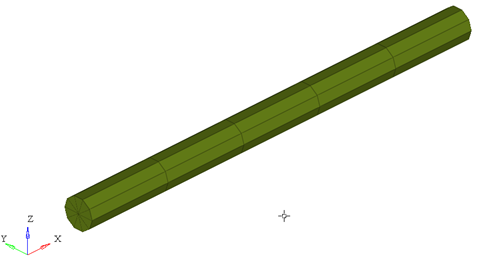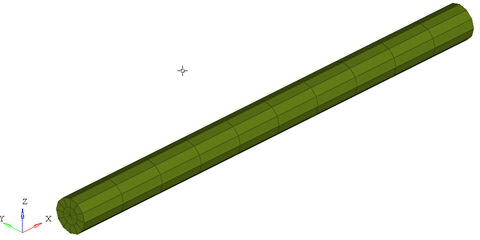# PBEAMC

Geometric Properties ElementPBEAMC lets you specify the geometric properties for an associated beam element of a circular cross section

## Format

<PBEAMC
id       = "integer"
mid      = "integer"
ri1      = "real"
ro1      = "real"
ri2      = "real"
ro2      = "real"
nf       = "real"
nx       = "integer"
nr       = "integer"
nt       = "integer"
ngx      = "integer"
ngr      = "integer"
ngt      = "integer"
graph    = "integer"
/>

## Attributes

id
Unique beam property identification number.
mid
Material property identification number.
ri1
Inner radius of the element cross section at node (1).
ro1
Outer radius of the element cross section at node (1).
ri2
Inner radius of the element cross section at node (2).
ro2
Outer radius of the element cross section at node (2).
nf
Specify a number greater than or equal to zero. The value of this attribute is used to reduce the bending stiffness of this element.
nx, nr, nt
Number of integration points in X, radial and tangential directions.
Default for nx is 5, nr is 4 and nt is 10.
ngx, ngr, ngt
Number of sub elements in X, radial and tangential directions. The default for ngx and ngr is 1, ngt is 10. 5.
graph
A post-processing flag that determines how this element is represented in the animation H3D. Default is 2.

## Example

The example demonstrates the definition of a PBEAMC property element.

<PBEAMC id="61" mid="1" ri1="50.0" ro1="60.0" ri2="3.0" ro2="2.0" nx="5" nr="4" nt="10" />

1. This type of property card is used to specify the geometric properties of the BEAMC and BEAM9 element. Each beam property element must have a unique identification number.
2. This property card defines the geometrical properties of the beam. The material properties of the beam are defined by the material specified by mid.
3. If only ri1 and ro1 are specified, MotionSolve assumes the cross section of the beam to be constant. However, if additionally, ri2 and ro2 are specified, the radii are varied linearly from node 1 to node 2 to represent the beam in the animation H3D.
4. For the beam element, nf can only be used with MAT6 to reduce the bending stiffness, that can related with the buckling stiffness.
5. graph is a post processing flag that determines how this element will be represented in the animation H3D file.
• graph = "0" implies that this element will not be represented in the H3D
• graph = "1" implies that this element will be represented as a line drawn between the two connecting nodes.
Note: When using graph="0" or graph="1", you will not be able to visualize the stress, strain or displacement contours. To do this, use graph="2" or graph="3".
• graph = "2" implies that the beam will be represented by 3D solid elements. This mode is useful when trying to visualize the stress/strain and displacement contours.
• graph = "3" implies that the beam is represented both as 3D solid elements as well as a line connecting the two nodes of the beam. This is useful when you need to visualize both the center line and the 3D representation of the beam.

When representing the beam as a solid, the arguments ngx, ngr and ngt determine the number of elements that are used to represent the beam in the animation H3D.ngx = ngr = 1; ngt = 1ngx = ngy = 2; ngt = 12

While increasing the ngx, ngr and ngt results in a better representation of the beam, it also increases the post-processing time taken by MotionSolve to write out the H3D. In addition, large values of ngx, ngr and ngt will increase the file size of the H3D considerably. Consider using the minimum values of these attributes that satisfy your visualization needs.